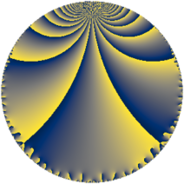# Properties

 Label 36.4.eLevel $36$ Weight $4$ Character orbit 36.e Rep. character $\chi_{36}(13,\cdot)$ Character field $\Q(\zeta_{3})$ Dimension $6$ Newform subspaces $1$ Sturm bound $24$ Trace bound $0$

# Learn more about

## Defining parameters

 Level: $$N$$ $$=$$ $$36 = 2^{2} \cdot 3^{2}$$ Weight: $$k$$ $$=$$ $$4$$ Character orbit: $$[\chi]$$ $$=$$ 36.e (of order $$3$$ and degree $$2$$) Character conductor: $$\operatorname{cond}(\chi)$$ $$=$$ $$9$$ Character field: $$\Q(\zeta_{3})$$ Newform subspaces: $$1$$ Sturm bound: $$24$$ Trace bound: $$0$$

## Dimensions

The following table gives the dimensions of various subspaces of $$M_{4}(36, [\chi])$$.

Total New Old
Modular forms 42 6 36
Cusp forms 30 6 24
Eisenstein series 12 0 12

## Trace form

 $$6q - 3q^{3} + 6q^{5} - 6q^{7} + 39q^{9} + O(q^{10})$$ $$6q - 3q^{3} + 6q^{5} - 6q^{7} + 39q^{9} + 51q^{11} + 12q^{13} - 180q^{15} - 222q^{17} + 30q^{19} - 120q^{21} + 210q^{23} - 3q^{25} + 648q^{27} + 456q^{29} + 48q^{31} - 603q^{33} - 1104q^{35} - 96q^{37} - 36q^{39} + 897q^{41} + 129q^{43} + 1494q^{45} + 522q^{47} - 225q^{49} - 1647q^{51} - 2208q^{53} - 216q^{55} - 645q^{57} + 453q^{59} - 402q^{61} + 1896q^{63} + 1110q^{65} - 213q^{67} - 198q^{69} + 120q^{71} + 750q^{73} + 921q^{75} + 1128q^{77} + 552q^{79} - 549q^{81} - 612q^{83} + 1188q^{85} - 1386q^{87} - 924q^{89} - 264q^{91} - 1998q^{93} - 2184q^{95} + 93q^{97} - 1854q^{99} + O(q^{100})$$

## Decomposition of $$S_{4}^{\mathrm{new}}(36, [\chi])$$ into newform subspaces

Label Dim. $$A$$ Field CM Traces $q$-expansion
$$a_2$$ $$a_3$$ $$a_5$$ $$a_7$$
36.4.e.a $$6$$ $$2.124$$ 6.0.6831243.2 None $$0$$ $$-3$$ $$6$$ $$-6$$ $$q+(-1-\beta _{2})q^{3}+(1-3\beta _{1}-2\beta _{2}-\beta _{3}+\cdots)q^{5}+\cdots$$

## Decomposition of $$S_{4}^{\mathrm{old}}(36, [\chi])$$ into lower level spaces

$$S_{4}^{\mathrm{old}}(36, [\chi]) \cong$$ $$S_{4}^{\mathrm{new}}(9, [\chi])$$$$^{\oplus 3}$$$$\oplus$$$$S_{4}^{\mathrm{new}}(18, [\chi])$$$$^{\oplus 2}$$